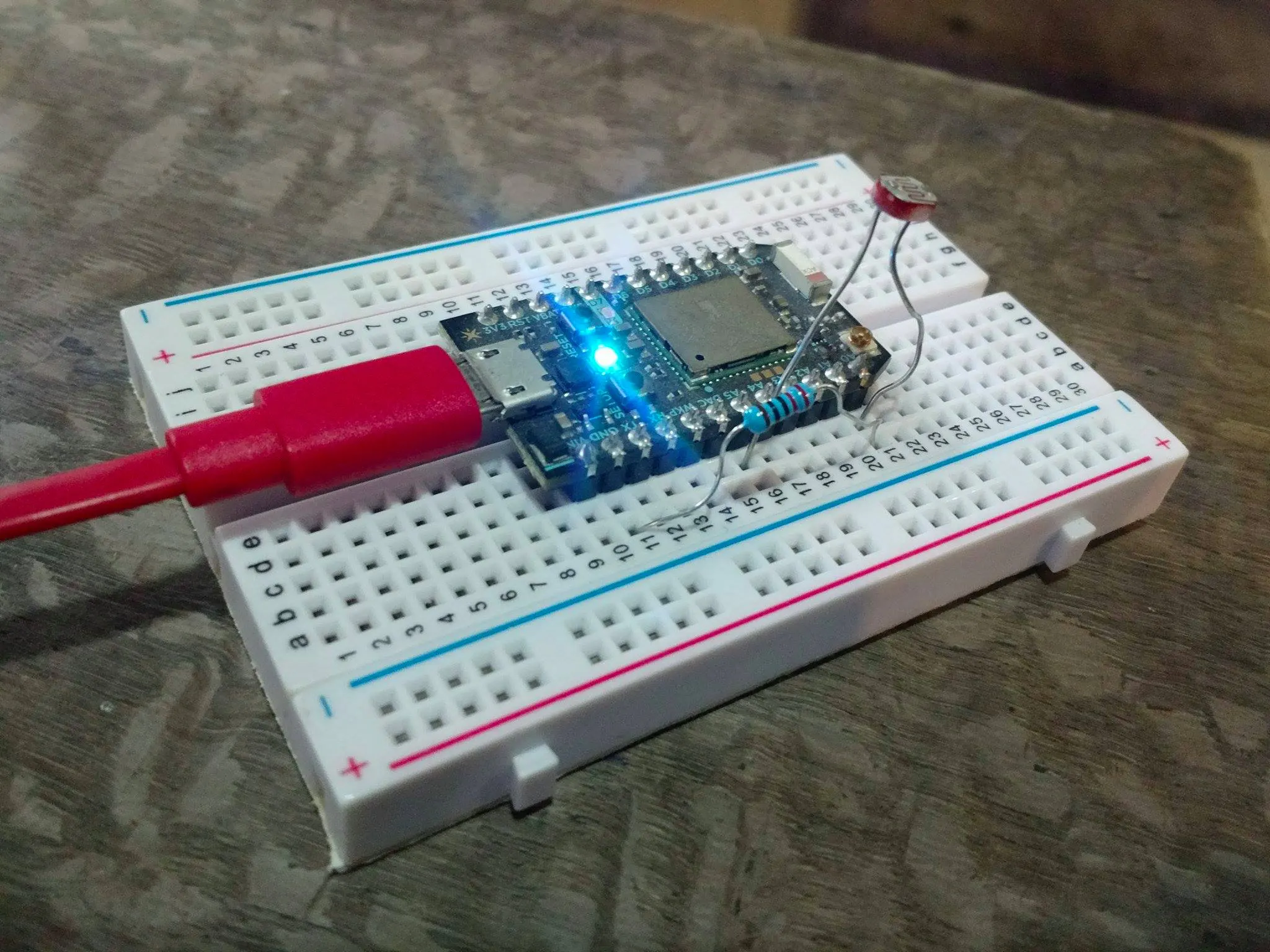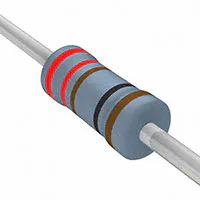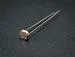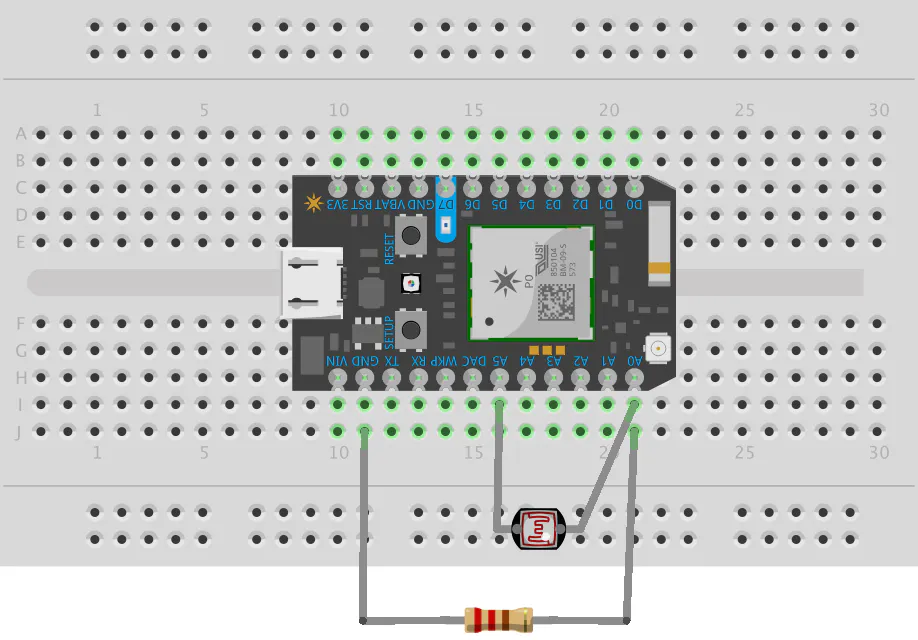# Light Detecting Email Sender

Receive an email about when the light is too strong on your device; nobody wants a heated device left directly under the sun. Photon + IFTTT

BeginnerFull instructions provided1 hour1,012## Things used in this project

### Hardware componentsParticle Photon
×1Resistor 221 ohm While I used a 220 Ohms resistor, you can use any resistor for this; a larger resistor (like 10K Ohms) will give you a wider range of values whereas a smaller resistor (like 220 Ohms) will give you a lower range of values.
×1Photo resistor
×1

## Schematics

### The Circuit Diagram

Build the circuit like the one given in the scheme diagram.## Code

### Untitled file

Arduino
This is the code for the photon you have to flash through build.particle.io to your device. Tweak around with delays for leds and intervals between each mails. You can even use an external LED instead of the inbuilt LED at D7. Also play around with the if condition for analog-value according to the safe limit you want to set.
```int led = D7; // This is where your can use can plug in a different LED. The other side should go to a resistor connected to GND.

int photoresistor = A0; // This is where your photoresistor is plugged in. The other side goes to the "power" pin (refer to diagram).

int power = A5; // This is the other end of your photoresistor. The other side is plugged into the "photoresistor" pin (above).
// The reason we have plugged one side into an analog pin instead of to "power" is because we want a very steady voltage to be sent to the photoresistor.
// That way, when we read the value from the other side of the photoresistor, we can accurately calculate a voltage drop.

int analogvalue; // Here we are declaring the integer variable analogvalue, which we will use later to store the value of the photoresistor.

void setup() {

// First, declare all of our pins. This lets our device know which ones will be used for outputting voltage, and which ones will read incoming voltage.
pinMode(led,OUTPUT); // Our LED pin is output (lighting up the LED)
pinMode(photoresistor,INPUT);  // Our photoresistor pin is input (reading the photoresistor)
pinMode(power,OUTPUT); // The pin powering the photoresistor is output (sending out consistent power)

// Next, write one pin of the photoresistor to be the maximum possible, so that we can use this for power.
digitalWrite(power,HIGH);

// We are going to declare a Particle.variable() here so that we can access the value of the photoresistor from the cloud.
Particle.variable("analogvalue", &analogvalue, INT);
// This is saying that when we ask the cloud for "analogvalue", this will reference the variable analogvalue in this app, which is an integer variable.

}

void loop() {

// check to see what the value of the photoresistor is and store it in the int variable analogvalue
delay(100);

if(analogvalue>=250){
digitalWrite(led, HIGH);
Spark.publish("Light_limit","Exceeded",60,PRIVATE);
delay(5000); //delay for LED
digitalWrite(led, LOW);
delay(10000); //10 second gap for each observation
// Add a delay to prevent getting tons of emails from IFTTT
}
}
```

## Credits

### Yatin Agarwal

0 projects • 1 follower
Founder at selfcure.co. A coding, chess, math, design, animation, music, cooking, and writing enthusiast.